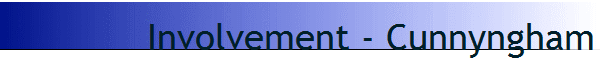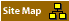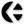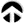Jon Cunnyngham was the first man, attending my first ever presentation, who understood what i presented: the principles of four dimensional dynamic logic.
He invited me to come and see him in his home, where we discussed what I was working on, and what he had been working on: a fuzzy logic in more dimensions.
His approach was based on the integration of the mathematical methods of matrix calculations and fuzzy logic analysis, using methods as used in physics combined with computational methods as used in psychiatry.
Therein, the calculations of the parameters and the variables are interchangeable: that means that both the variables and the parameters are calculated mirroring each other.

John Cunnyngham had done fundamental national budget econometric computations, using the largest computer available in the United States to do bulk computer operations.
As a result he had come to understand how a mathematical error and computational error all compound to the unreliability and uncertainty of the result.
As a result he had also realised that it was necessary to do not only testing calculations but also corrective computations.
This had led to his interest in fuzzy logic and the approach of what we can now call inverse calculation.

This is the same kind of calculation which is used per by engineers in calculating the interplay between a River and its Bedding.
For one step of the calculation, the bedding of the River is considered to be fixed, and the (effect on the) flow of the River is computed.
For the next step, the flow of the River stream is assumed as a given, and the effect on transforming the boundary is computed.
The computation itself consist of ‘stepping’ through a series of computations on the one side and the other side of the boundary, to hopefully end up with a realistic result.

In this same way it can be seen that it is possible to use the relationship in an equation between 1) the values, 2) the processes, 3) the transformative operators, and 4) the equation sign.
In fact, the step-wise computational method of the calculation of the River bedding showed that is possible to compute ‘two different sides of the equation sign’ and thus in fact operate with two different ‘forms’ of the equation sign in the computation.
If the dimensional state (for example the effect of scaling) would be included in the computation, then there would be a third level in which the variability of the parameters of the calculation would need to be calculated themselves.
What then would become explicit is that the equation sign for each of these levels of equations would refer to a different kind of state (dimension) of the computation, and of the realisation.

In fact in the calculation of the River bedding the equation sign would refer to the fixed state of matter, and thus would be of a physical nature dots
In the second stage of the equation, of the calculation of the flow of the River, the equation sign would be referring to the dynamic process, and would be the equivalent of a chemical calculation.
At the third level, in computing the scaling characteristics of the model, the equation sign would refer to a multiples state of transformation of the equation itself.
At the fourth level we would need to account for the fact that the equation sign is in fact the representation of our involvement in the mathematical computation.

This relationship is made explicit in system theory.
There, there are four levels of consideration which are considered at the same time.
These are known as perspective of 1) state, 2) process, 3) transformation and 4) integration.
For a detailed description of the way this works, please read the book General  system theory by Ludwig von Bertalannfy.

MataThematics, and the 4D Dimensional Logic, this use the same principles as system theory (although it was derived from a different basis.).
Arthur M Young has described the relationship between these four different dimensions, in his book ”the Geometry of Meaning”.
He described the relationship between 1) position, 2) velocity, 3) acceleration, and 4) integration.
These are the four elements that we need to consider in the calculus of involvement.

The calculation method of Jon Cunnyngham probably comes closest to the intent of MetaThematics.
in using the approach of psychiatry, Jon Cunnyngham interchanged the computation of the variables with the computation of the parameters.
This is equivalent to the so-called weighing-functions of algebra, where the parameters are adjusted, to check the validity of the outcomes of the variables in the computation in, for example, engineering or physics.
What we see there, is the attunement/calibration of the computation (to suit the purpose of the computing itself) with respect to a specific desired or anticipated outcome.

This is related to the “Hand-Glove” approach used by Roberto Renout.
He used this in astrology, to compute the correlation between the exact astrological computation and the predictions/interpretations by his astrological program.
This makes use of an element of astrology known as “rectification”.
This is a method for correction of the precise moment of birth, by computing the exact moment of characteristic turning-points in life.

See: Involvement: Renout, and www.hypermandala.com.

This too is similar to what is done in psychiatric computation, were the calculations are ‘doctored’, in order to get a more fitting outcome.
Again, this is based on the standard mathematical principles of curve fitting analysis and function weighing analysis, which are commonplace mathematical analytical practice.
In this case it means that the calculations are inverted, with respect to the role of the parameters and the variables.
Again, this is very similar to the way the calculation is done in the interplay between a river and its bedding.

John Cunnyngham realised that he would need this kind of flexible adaptation of the equations.
In fact, out of this observation of the interchangeability of variables and parameters, he came up with a much deeper insight into mathematics.
He realised that there is a more abstract level of mathematics, which here we have called the Operator Algebra level mathematics, were likewise different forms of logic can be interchanged.
It means that the different forms of mathematics are as interchangeable with each other as are the variables and the parameters in the standard mathematical equations (in smoothing/weighing analysis).

### Context defined mathematics

With this understanding, Jon Cunnyngham was able to come to this deeper form of mathematics, with a much deeper level of relevance.
As he described it, there is a level of mathematics, which is much more abstract, which is defined by the conditions in which it is applied.
This is typical for any 3rd Order computation (as it is known in system theory); were we are dealing with the characteristic system boundary transition.
This is the equivalent of the type of mathematics operated in dimensional analysis, where the definition of the calculation itself needs to be defined.

For Jon Cunnyngham it meant that he came up with a much more fundamental form of mathematics.
Depending on the context to which he applied it, out would come the traditional forms of calculation methods as they were customarily applied.
It meant that the various forms of calculations, as used in psychiatry and in science, are merely aspects of this much deeper form of computation.
Compare this also with the notes on the work of Uri Fidelman, demonstrating that the equations of science are in fact the representations of the way our mind works.

In the work of Jon Cunnyngham it meant that there is one deeper, more amorphous, form of mathematics: this is what I would call MetaThematics.
Defining the context for that more abstract form of mathematics will define different forms/branches/types/logic of mathematics which can be used.
it means that we should not concern ourselves with those various kinds of mathematics, each defined by/within their rather specific/limited modes of context.
instead we should realise that there is a fundamental form of mathematics, that there is a separate level of mathematics dealing with the definition and redefinition of the context, and that only then can we can come to mathematics which will give more specific outcomes to our computations.

This principle i have described in an early paper entitled "Patterns & Processes, Principles & Essences".
It deals with the difference in the relationship of Values (Predictables), Variables, Operators, at the Equation sign.
However, at that time, at that level, it was described in terms of a specific outcome, or purpose, of the mathematical equations.
At that level, the 1) parameters, 2) the processes, 3) the principles and 4) the essence (involvement) referred to the difference types of relevance of the computations that we do.

### Geogebra and mystic experience

In the work of Jon Cunnyngham this was made explicit by the way he correlated the algebraic and the geometric characteristics of the computations.
He showed this in the very explicit superposition of the atomic spectrum diffraction grid as photographed for a specific atomic element.
He correlated that with traditional mystic geometric symbol systems, such as the “Flower of Life” and the “Kabalaw”.
Therein he was able to show that the traditional Mystic system for description of Essences are in fact paradigms or metaphors for the way that we can operate the level of mathematical contextual calculations.

That means that there is a direct correlation between geometry, algebra, mystic symbolism, and this deeper level of calculation/computation.
It also means that we must explicitly account for our context, but also, explicitly, for our own involvement.
It is only when we precision our own specific involvement, that we can give value and meaning to the calculation and thus to the outcome.
But also, this means that we thereby must be able to define and describe our specific form of involvement compared to that of anyone else.

This is where we then see the conjunction of algebra, geometry, mysticism, and our own direct experience in realisation.
It is clear from the work of Jon Cunnyngham, that we cannot be content with the use of the traditional forms of mathematics as taught in academia.
Instead, in fact, we must use this deeper form of mathematics, which is in fact equivalent with the traditional mystical symbolic systems.
As intermediate, or interface, between these different perspectives, the approach of system theory can bridge the seeming gap between them.

Thus, by the work of Jon Cunnyngham, we can see that we have this deeper level of mathematics which corresponds with our own choice in involvement.
This insight was based on the realisation of the interchangeability of the computations of atomic physics, econometrics, the weather bureau and psychiatry.
Which shows that we are actually dealing with the same kind of matrix mathematics, but now applied to our own specific mode of involvement.
This is why the work of John Cunningham is an excellent example of one of the key elements that we need in our understanding for the mathematics of involvement.

http://jon.cunnyngham.net[Welcome] [Core Concepts] [Topics] [Participants] [Publications] [Research] [Projects]